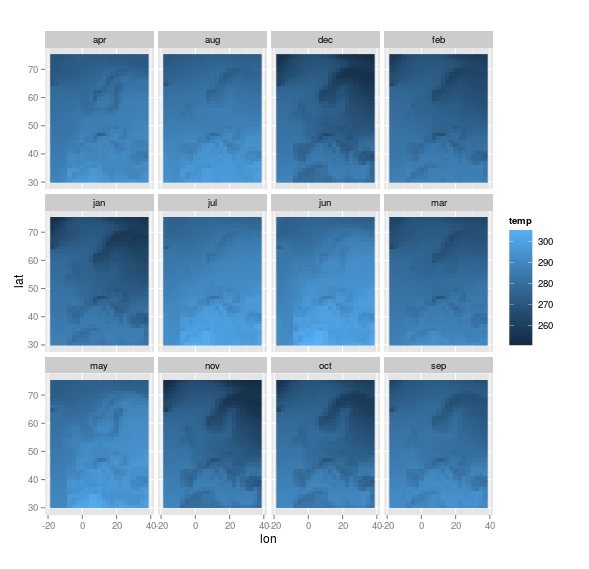Want to share your content on R-bloggers? click here if you have a blog, or here if you don't.

Mid november, a nice workshop on big data and environment will be organized, in Argentina,We will talk a lot about climate models, and I wanted to play a little bit with those data, stored on http://dods.ipsl.jussieu.fr/mc2ipsl/.

Since Ewen (aka @3wen) has been working on those datasets recently, he kindly told me how to read those datasets (in some ncdf format). He did show me interesting functions, and did share with me some lines of codes. I will mention some of them in a brief post, because I’ve been impressed to see how memory issues were not a big deal, here… But first of all, we need half a dozen packages

```> library(ncdf)
> library(lubridate)
> library(dplyr)
> library(stringr)
> library(tidyr)
> library(ggplot2)```

Then i will download one of those files, from January 2000 till December 2012.

```> download.file("http://dods.ipsl.jussieu.fr/
mc2ipsl/CMIP5/output1/IPSL/IPSL-CM5A-MR/historicalNat/day/atmos/day/r1i1p1/latest/tas/tas_day_IPSL-CM5A-MR_historicalNat_r1i1p1_20000101-20121231.nc",destfile="temp.nc")
```

Those file are not huge. But big.

```> file.size("temp.nc")
 390965452```

That’s almost a 400Mo file. For one single file (and there are hundreds on those servers). Now, if we ‘read’ that file, in R, we have a rather small R object,

```> nc <- open.ncdf("temp.nc")
> object.size(nc)
155344 bytes```

That’s only 150Ko… One out of 2516 compared with the file online. Somehow, we only extract partial information here. We will scan the file completely later on… We can already create some variables that will be used later on, such as the date, the latitude, the longitude, etc

```> lon <- get.var.ncdf(nc,"lon")
> (nlon <- dim(lon))
 144
>  lat <- get.var.ncdf(nc,"lat")
> nlat <- dim(lat)
> time <- get.var.ncdf(nc,"time")
> tunits <- att.get.ncdf(nc, "time", "units")
> orig <- as.Date(ymd_hms(str_replace(
tunits\$value, "days since ", "")))
> dates <- orig+time
> ntime <- length(dates)```

Here we have the range of latitude and longitude in the dataset, as well as the date. Now it is time to read the file. Based on the starting date, and asking only for temperature

```> commencement <- ind_conserver
> dname="tas"```

we can red the file

```> tab_val <- get.var.ncdf(nc, dname, start=c(1,1,commencement))
> dim(tab_val)
  144  143 4745
> object.size(tab_val)
781672528 bytes```

The file is big now, a bit less that 800Mo.

```> fill_value <- att.get.ncdf(nc, dname, "_FillValue")
> tab_val[tab_val == fill_value\$value] <- NA```

Let us now summarize all the information in nice data frames, that will be used to generate graphs

```> tab_val_vect <- as.vector(tab_val)
> tab_val_mat <- matrix(tab_val_vect, nrow =
nlon * nlat, ncol = ntime)
> lon_lat <- expand.grid(lon, lat)
> lon_lat <- lon_lat %>% mutate(Var1 =
as.vector(Var1), Var2 = as.vector(Var2))```

Note that the file is still big (the same size as the previous file, here we just reshape the dataset)

```> res <- data.frame(cbind(lon_lat,
tab_val_mat))
> object.size(res)
782496048 bytes```

Here is the dimension of our dataset

```> dim(res)
 20592  4747```

We can give understandable names to variables

```> noms <- str_c(year(dates_conserver),
+ month.abb[month(dates_conserver)] %>%
tolower, sprintf("%02s", day(dates_conserver)),
sep = "_")
> names(res) <- c("lon", "lat", noms)```

Now, let us focus on the ploting part. If we focus on Europe, we need to filter the dataset

```> res <- res %>%
mutate(lon = ifelse(lon >= 180, yes = lon -
360, no = lon)) %>%
filter(lon < 40, lon > -20, lat < 75,
lat > 30) %>%
gather(key, value, -lon, -lat) %>%
separate(key, into = c("year", "month",
"day"), sep="_")```

Let use filter, with only years from 1986 till 2005

```> temp_moy_hist <- res %>%
+ filter(year >= 1986, year <= 2005) %>%
+ group_by(lon, lat, month) %>%
+ summarise(temp = mean(value, na.rm = TRUE)) %>%
+ ungroup```

Now we can plot it. With the temperature in Europe, per month.

```> ggplot(data = temp_moy_hist,
aes(x = lon, y = lat, fill = temp)) +
geom_raster() + coord_quickmap() +
facet_wrap(~month)```Actually, it is possible to add contour lines of European countries,

```> download.file(
"http://freakonometrics.free.fr/carte_europe_df.rda",destfile="carte_europe_df.rda")

If we aggregate all the month, and get only one graph, we have

```> ggplot() +
geom_raster(data = temp_moy_hist,
aes(x = lon, y = lat, fill = temp)) +
geom_polygon(data = carte_pays_europe,
aes(x=long, y=lat, group=group), fill = NA,
col = "black") +
coord_quickmap(xlim = c(-20, 40),
ylim = c(30, 75))```I will spend some time now to analyze the maps, but I have been really impressed by those functions, that can partially read a ‘big’ dataset, to extract some information, and then we can read the file to create a dataset that can be used to get a graph.